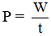# Physics

### Chapter : 3. Work and Energy

#### Examples of Power

Example : 9
A weight lifter lifted a load of 100 kg to a height of 3 m in 10 s. Calculate the following:
(i) amount of work done
(ii) power developed by him
Solution. (i) Work done is given by
W = F . s
Here, F = mg = 100 × 10 = 1000 N
W = 1000 N × 3 m = 3000 joule
(ii) Now,, where W = 3000 J and t = 10 s
∴ P = 3000J/10s = 300 W

Example : 10
A water pump raises 60 liters of water through a height of 20 m in 5 s. Calculate the power of the pump. (Given: g = 10 m/s2, density of water = 1000 kg/m3)
Solution. Work done, W = F.s...(1)
Here, F = mg ...(2)
But, Mass = volume × density
Volume = 60 liters = 60 × 10–3 m3
Density = 1000 kg/m3
∴ Mass , m = (60 × 10–3 m3) × (1000 kg/m3) = 60 kg
∴ Equation (2) becomes
F = 60 kg × 10 m/s2 = 600 N
Now, W = F . s = 600 N × 20 m = 12000 J
Power == 12000J/5s = 2400 W

Example : 11
A woman pulls a bucket of water of total mass 5 kg from a well which is 10 m deep in 10 s. Calculate the power used by her.
Solution. Given that m = 5 kg; h = 10 m; t = 10 s, g = 10 m/s2
Now,